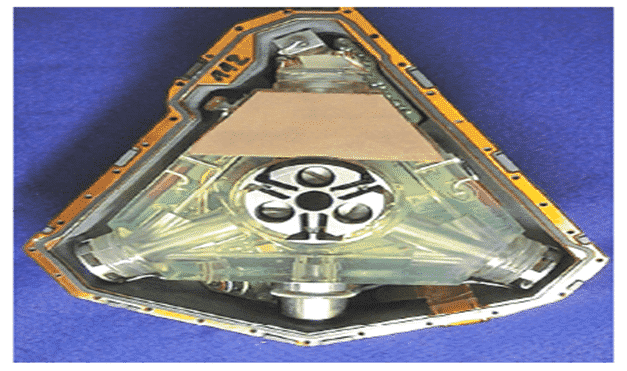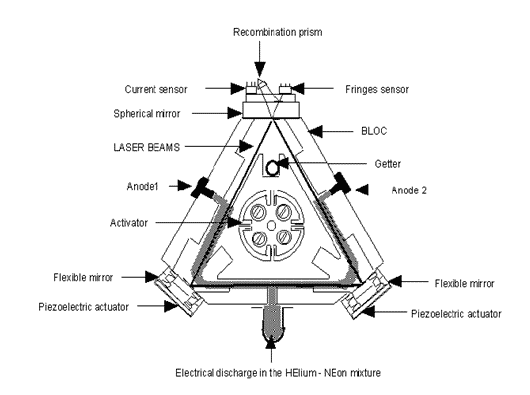The Ring laser gyro (RLG) functions in the following way :

• The laser generates a very coherent beam of light. This concludes that since the source is same, the generated photons are at one specific frequency. The beam is split into two beams that travel the same path but in opposite directions: one clockwise and the other counter-clockwise.
• The beams are recombined and sent to the output detector. In the absence or rotation, the path lengths will be the same and the output will be the total constructive interference of the two beams.
• If the apparatus rotates, there will be a difference in the path lengths traveled by the two beams, resulting in a net phase difference and destructive interference. The net signal will vary in amplitude depending on the phase shift, therefore the resulting amplitude is a measurement of the phase shift, and consequently, the rotation rate.
• Doppler shift in sound frequency. An inbound train whistle changes from high to low pitch as the train goes by and then outbound relative to the observer who is standing still waiting to cross the tracks. Same theory applies to light.Advantages of Ring Laser Gyro are as follows :

• It gives digital output with angle increments
• It has very high sensitivity
• It has stable input axis
• easy detection over a wide range of measurement (from 10-3”/h to thousands of “ i s )
• It is insensitive to accelerations as it has no moving parts. Thus the chances of errors due to acceleration is nullified.
• It is very reliable.

Sources of error in the Ring Laser Gyro:

Ideally, the response curve of the RLG is linear. The frequency difference is proportional to the rotation rate. Any effect which cause a deviation from this straight line must be considered as a source of error as it may limit the performance of the RLG as an inertial sensor. In the conventional two mode active laser gyro there are three main types of errors :

• scale factor variations
• null shift, and
• mode locking